CBSE Class 10 Sample Paper for 2020 Boards - Maths Standard

Class 10
Solutions of Sample Papers for Class 10 Boards

## If the sum of first m terms of an AP is the same as the sum of its first n terms, show that the sum of its first (m + n) terms is zero.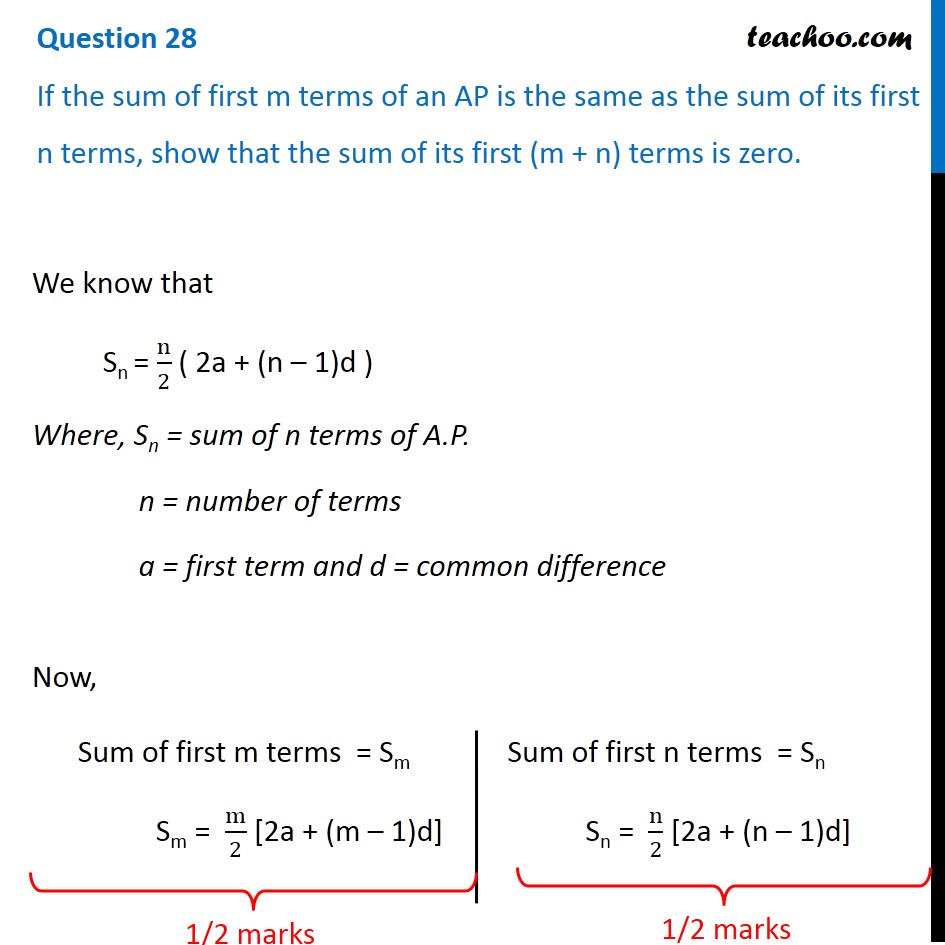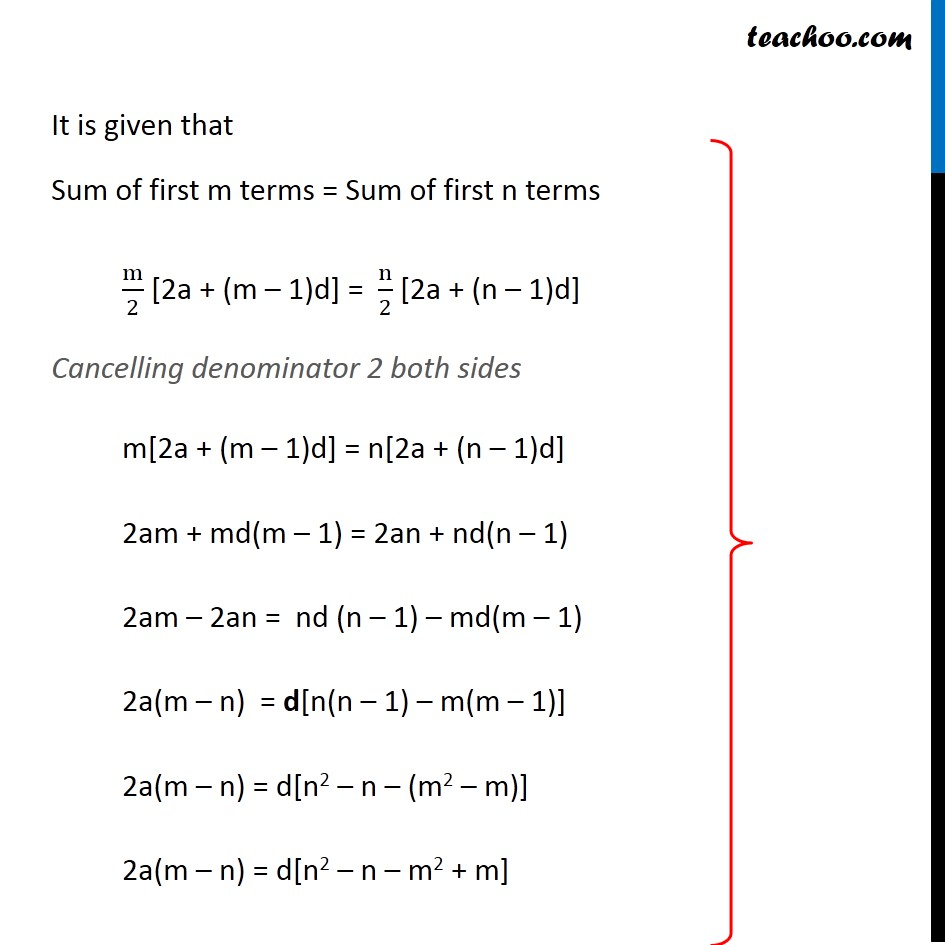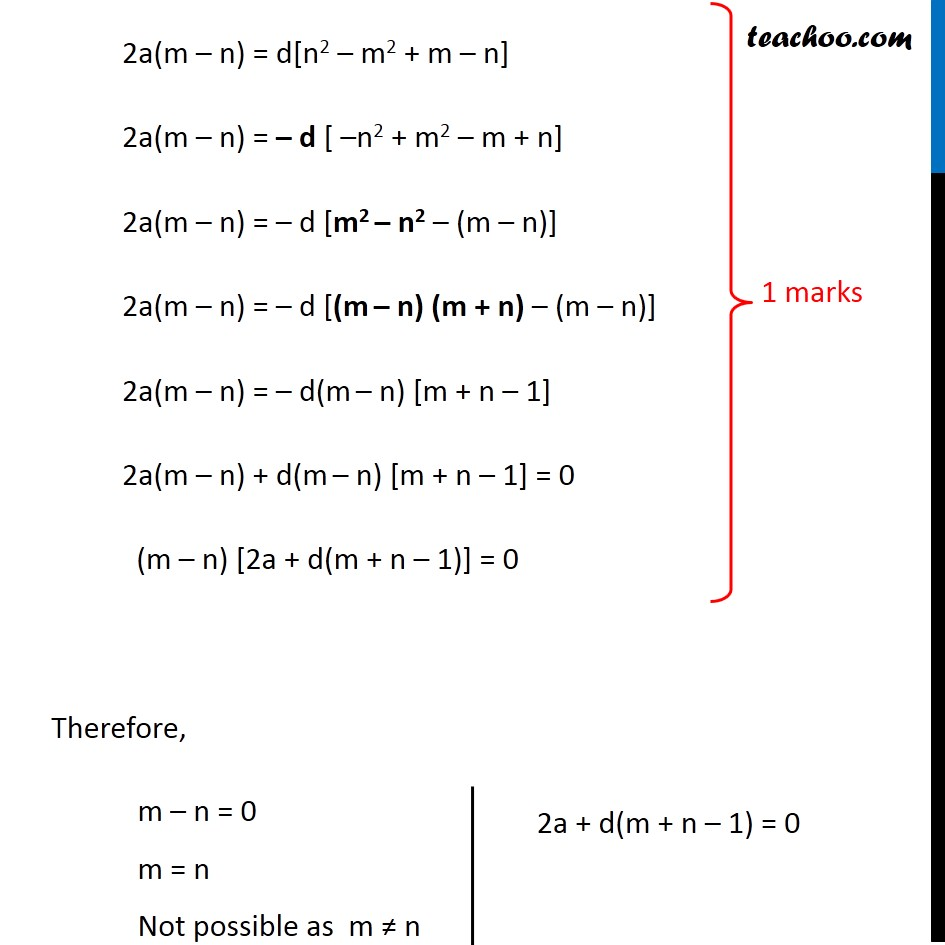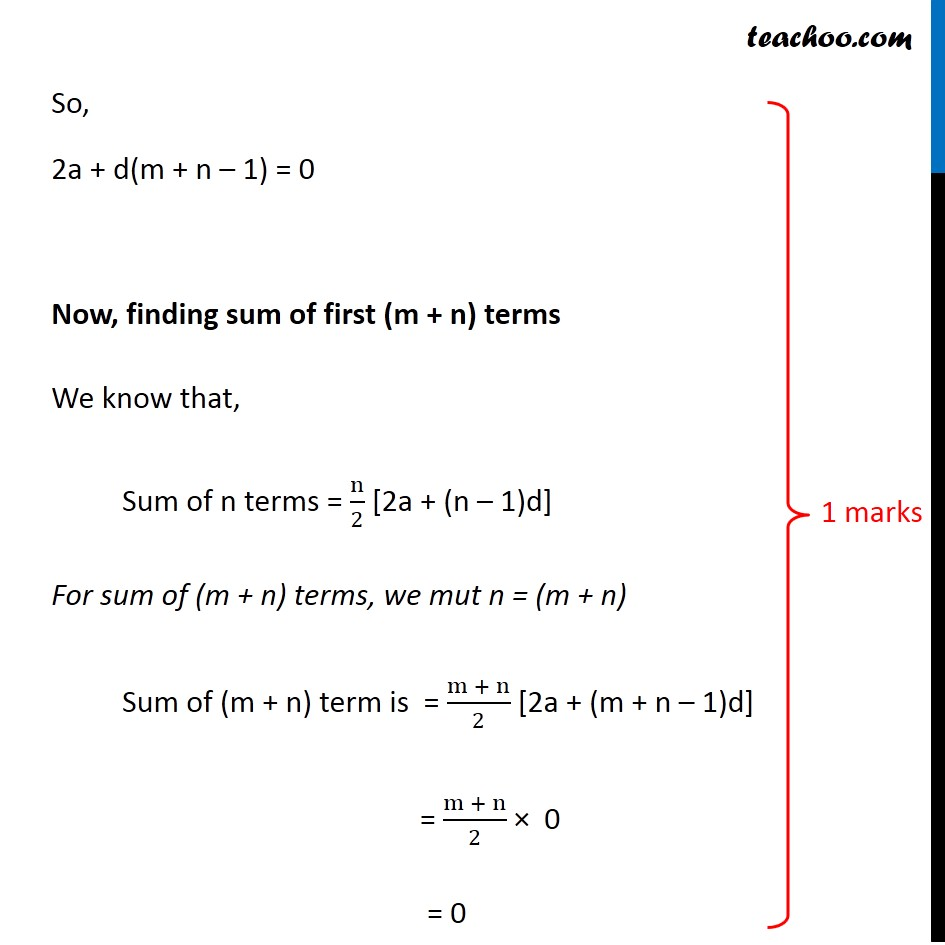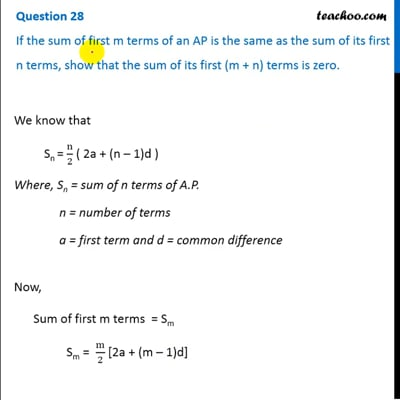This video is only available for Teachoo black users

Note : This is similar to Ex 9.2, 10 of NCERT – Chapter 9 Class 11

Learn in your speed, with individual attention - Teachoo Maths 1-on-1 Class

### Transcript

Question 28 If the sum of first m terms of an AP is the same as the sum of its first n terms, show that the sum of its first (m + n) terms is zero. We know that Sn = n/2 ( 2a + (n – 1)d ) Where, Sn = sum of n terms of A.P. n = number of terms a = first term and d = common difference Now, Sum of first m terms = Sm Sm = m/2 [2a + (m – 1)d] Sum of first n terms = Sn Sn = n/2 [2a + (n – 1)d] It is given that Sum of first m terms = Sum of first n terms m/2 [2a + (m – 1)d] = n/2 [2a + (n – 1)d] Cancelling denominator 2 both sides m[2a + (m – 1)d] = n[2a + (n – 1)d] 2am + md(m – 1) = 2an + nd(n – 1) 2am – 2an = nd (n – 1) – md(m – 1) 2a(m – n) = d[n(n – 1) – m(m – 1)] 2a(m – n) = d[n2 – n – (m2 – m)] 2a(m – n) = d[n2 – n – m2 + m] 2a(m – n) = d[n2 – m2 + m – n] 2a(m – n) = – d [ –n2 + m2 – m + n] 2a(m – n) = – d [m2 – n2 – (m – n)] 2a(m – n) = – d [(m – n) (m + n) – (m – n)] 2a(m – n) = – d(m – n) [m + n – 1] 2a(m – n) + d(m – n) [m + n – 1] = 0 (m – n) [2a + d(m + n – 1)] = 0 Therefore, m – n = 0 m = n Not possible as m ≠ n 2a + d(m + n – 1) = 0 So, 2a + d(m + n – 1) = 0 Now, finding sum of first (m + n) terms We know that, Sum of n terms = n/2 [2a + (n – 1)d] For sum of (m + n) terms, we mut n = (m + n) Sum of (m + n) term is = (m + n)/2 [2a + (m + n – 1)d] = (m + n)/2 × 0 = 0 ∴ Sum of (m + n) term is 0 Hence proved